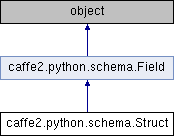Caffe2 - Python API A deep learning, cross platform ML framework
caffe2.python.schema.Struct Class Reference
Inheritance diagram for caffe2.python.schema.Struct:## Public Member Functions

def __init__ (self, fields)

def get_children (self)

def field_names (self)

def field_types (self)

def field_blobs (self)

def all_scalars (self)

def has_blobs (self)

def clone (self, keep_blobs=True)

def __contains__ (self, item)

def __len__ (self)

def __getitem__ (self, item)

def get (self, item, default_value)

def __getattr__ (self, item)

def __setattr__ (self, key, value)

def __sub__ (self, other)Public Member Functions inherited from caffe2.python.schema.Field
def __init__ (self, children)

def clone_schema (self)

def field_names (self)

def field_types (self)

def field_blobs (self)

def all_scalars (self)

def has_blobs (self)

def clone (self, keep_blobs=True)

def slice (self)

def __eq__ (self, other)

def __repr__ (self)

fields

## Detailed Description

```Represents a named list of fields sharing the same domain.
```

Definition at line 279 of file schema.py.

## Constructor & Destructor Documentation

 def caffe2.python.schema.Struct.__init__ ( self, fields )
```fields is a list of tuples in format of (name, field). The name is
a string of nested name, e.g., `a`, `a:b`, `a:b:c`. For example

Struct(
('a', Scalar()),
('b:c', Scalar()),
('b:d:e', Scalar()),
('b', Struct(
('f', Scalar()),
)),
)

is equal to

Struct(
('a', Scalar()),
('b', Struct(
('c', Scalar()),
('d', Struct(('e', Scalar()))),
('f', Scalar()),
)),
)
```

Definition at line 283 of file schema.py.

## Member Function Documentation

 def caffe2.python.schema.Struct.__add__ ( self, other )
```Allows to merge fields of two schema.Struct using '+' operator.
If two Struct have common field names, the merge is conducted
recursively. Here are examples:

Example 1
s1 = Struct(('a', Scalar()))
s2 = Struct(('b', Scalar()))
s1 + s2 == Struct(
('a', Scalar()),
('b', Scalar()),
)

Example 2
s1 = Struct(
('a', Scalar()),
('b', Struct(('c', Scalar()))),
)
s2 = Struct(('b', Struct(('d', Scalar()))))
s1 + s2 == Struct(
('a', Scalar()),
('b', Struct(
('c', Scalar()),
('d', Scalar()),
)),
)
```

Definition at line 468 of file schema.py.

 def caffe2.python.schema.Struct.__getitem__ ( self, item )
```item can be a tuple or list of ints or strings, or a single
int or string. String item is a nested field name, e.g., "a", "a:b",
"a:b:c". Int item is the index of a field at the first level of the
Struct.
```

Definition at line 417 of file schema.py.

 def caffe2.python.schema.Struct.__sub__ ( self, other )
```Allows to remove common fields of two schema.Struct from self by
using '-' operator. If two Struct have common field names, the
removal is conducted recursively. If a child struct has no fields
inside, it will be removed from its parent. Here are examples:

Example 1
s1 = Struct(
('a', Scalar()),
('b', Scalar()),
)
s2 = Struct(('a', Scalar()))
s1 - s2 == Struct(('b', Scalar()))

Example 2
s1 = Struct(
('b', Struct(
('c', Scalar()),
('d', Scalar()),
))
)
s2 = Struct(
('b', Struct(('c', Scalar()))),
)
s1 - s2 == Struct(
('b', Struct(
('d', Scalar()),
)),
)

Example 3
s1 = Struct(
('a', Scalar()),
('b', Struct(
('d', Scalar()),
))
)
s2 = Struct(
('b', Struct(
('c', Scalar())
('d', Scalar())
)),
)
s1 - s2 == Struct(
('a', Scalar()),
)
```

Definition at line 509 of file schema.py.

 def caffe2.python.schema.Struct.get ( self, item, default_value )
```similar to python's dictionary get method, return field of item if found
(i.e. self.item is valid) or otherwise return default_value

it's a syntax suger of python's builtin getattr method
```

Definition at line 442 of file schema.py.

The documentation for this class was generated from the following file: